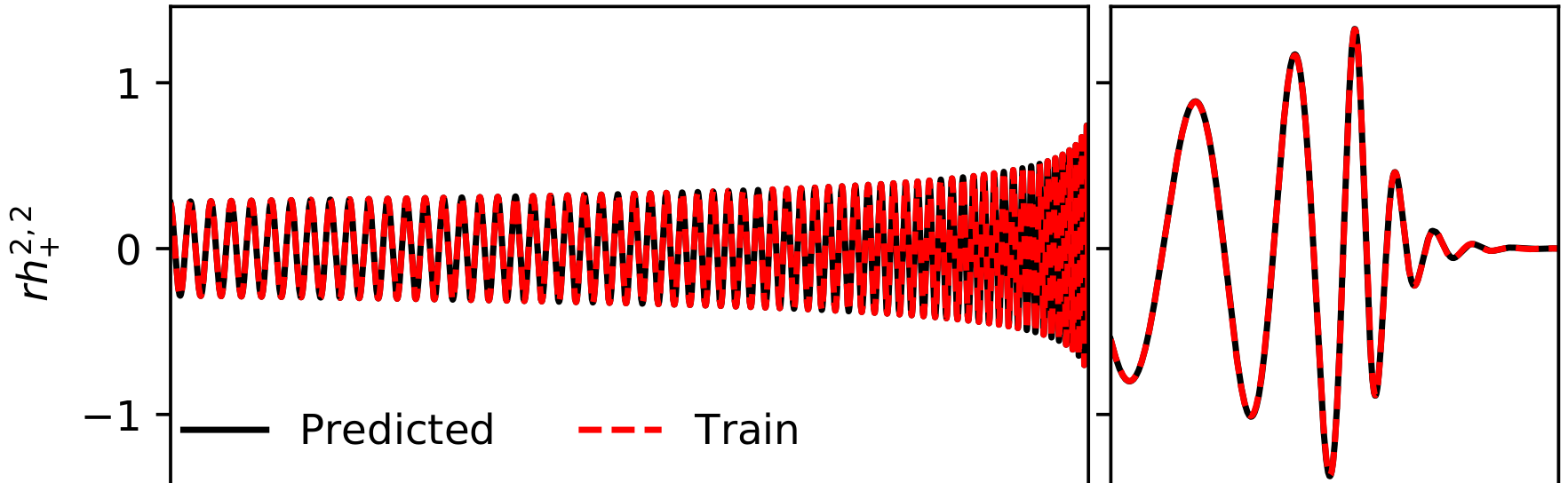# BHPTNRSurrogate

Get the code!

Python code to evaluate gravitational waveform surrogate models trained on waveform data generated by point-particle black hole perturbation theory (BHPT) and calibrated to numerical relativity (NR) in the comparable mass regime.## Model Details

The BHPTNRSurrogate package provides access to a surrogate gravitational waveform model. Currently, this package supports one model, BHPTNRSur1dq1e4, for non-spinning black hole binary systems with mass-ratios varying from 3 to $10^4$. The “raw” surrogate model is trained on waveform data generated by point-particle black hole perturbation theory (ppBHPT) and defined as, \begin{align} h_{\tt S}(t, \theta, \phi) = \sum^{\infty}_{\ell=2} \sum_{m=-\ell}^{\ell} h_{\tt S}^{\ell,m}(t) ~^{-2}Y_{\ell m}(\theta, \phi) \,, \end{align} where $^{-2}Y_{\ell m}$ are the spin$=-2$ weighted spherical harmonics. The surrogate model provides fast evaluations for the modes, $h_{\tt S}^{\ell,m}$. Over 50 available $m > 0$ modes are included, including up to $\ell=10$, while the $m<0$ modes $h^{\ell, -m} = (-1)^{\ell} h^{\ell,m}{}^*$ can be deduced from the $m>0$ modes.

By default, the BHPTNRSurrogate package will return rescaled waveform modes, \begin{align} h^{\ell,m}_{\tt S, \alpha}(t ; q)= {\alpha_{\ell}} h^{\ell,m}_{\tt S}\left( t \beta;q \right) \,, \end{align} where the calibration parameters, $\alpha_{\ell}(q)$ and $\beta(q)$, are tuned to NR simulations. We expect most users of this package will want the NR-tuned model. To generate point-particle perturbation theory waveforms without any tuning to NR set $\alpha_{\ell} = \beta = 1$ in the function slog_surrogate.

Model details can be found in paper 1 (see citations below).

## Getting the code

The latest development version will always be available from the project git repository:

git clone https://github.com/BlackHolePerturbationToolkit/BHPTNRSurrogate.git


This code, in turn, is based on the EMRISurrogate package, which is no longer actively maintained.

## Requirements, Installation, and Usage

For details on these topics check out the README and the Jupyter notebook.

## Known problems

Known bugs are recorded in the project bug tracker

This code is distributed under the MIT License. Details can be found in the LICENSE file.

## Authors

Scott Field, Tousif Islam, Gaurav Khanna, Nur Rifat, Vijay Varma

## Citation guideline

If you make use of any module from the Toolkit in your research please acknowledge using “This work makes use of the Black Hole Perturbation Toolkit”. If you make use of the surrogate model, BHPTNRSur1dq1e4, please cite Paper 1:

@article{islam2022surrogate,
title={Surrogate model for gravitational wave signals from non-spinning, comparable-to large-mass-ratio black hole binaries built on black hole perturbation theory waveforms calibrated to numerical relativity},
author={Islam, Tousif and Field, Scott E and Hughes, Scott A and Khanna, Gaurav and Varma, Vijay and Giesler, Matthew and Scheel, Mark A and Kidder, Lawrence E and Pfeiffer, Harald P},
journal={arXiv preprint arXiv:2204.01972},
year={2022}
}


as well as Paper 2, which developed the original software infrastructure that the BHPTNRSurrogate package is based on:

@article{rifat2019surrogate,
title={A Surrogate Model for Gravitational Wave Signals from Comparable-to Large-Mass-Ratio Black Hole Binaries},
author={Rifat, Nur EM and Field, Scott E and Khanna, Gaurav and Varma, Vijay},
journal={arXiv preprint arXiv:1910.10473},
year={2019}
}